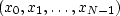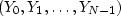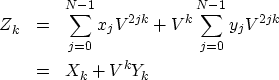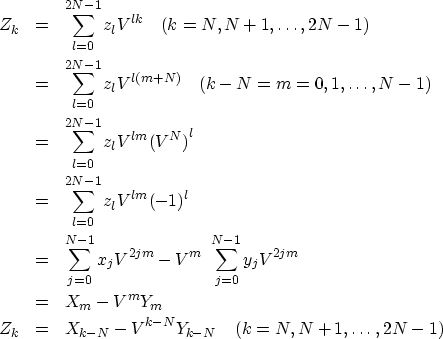Next: References Up: Discrete Fourier transform Previous: Examples of 2-D FT

# HOW FAST FOURIER TRANSFORM WORKS

A basic building block in the fast Fourier transform is called doubling.'' Given a seriesand its sampled Fourier transform,and another seriesand its sampled Fourier transform,there is a trick to find easily the transform of the interlaced double-length series(20)

The process of doubling is used many times during the computing of a fast Fourier transform. As the word doubling" might suggest, it will be convenient to suppose that N is an integer formed by raising 2 to some integer power. Suppose N = 8 = 23. We begin by dividing our eight-point series into eight separate series, each of length one. The Fourier transform of each of the one-point series is just the point. Next, we use doubling four times to get the transforms of the four different two-point series (x0, x4), (x1, x5), (x2, x6), and (x3, x7). We use doubling twice more to get the transforms of the two different four-point series (x0, x2, x4, x6) and (x1, x3, x5, x7). Finally, we use doubling once more to get the transform of the original eight-point series.It remains to look into the details of the doubling process. Let(21) (22)
By definition, the transforms of two N-point series are(23) (24)
Likewise, the transform of the interlaced seriesis(25)
To make Zk from Xk and Yk, we require two separate formulas, one for k = 0, 1,, N -1, and the other for k = N, N + 1,, 2N - 1. Start from the sum(26)
and then split the sum into two parts, noting that xj multiplies even powers of V, and yj multiplies odd powers:(27) (28)
We obtain the last half of the Zk by(29) (30) (31) (32) (33) (34) (35)

The subroutine ftu()does not follow this analysis in detail.

If you would like some industrial grade FFT programs, search the web for "prime factor FFT".Next: References Up: Discrete Fourier transform Previous: Examples of 2-D FT
Stanford Exploration Project
10/21/1998